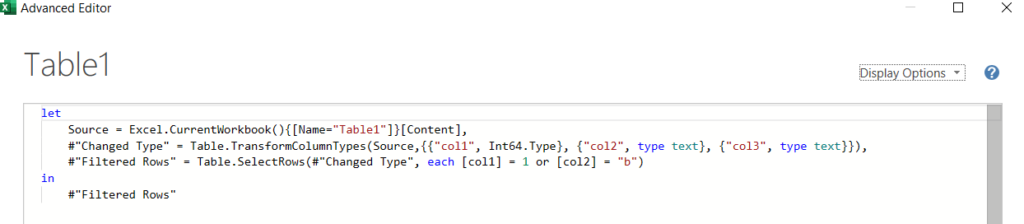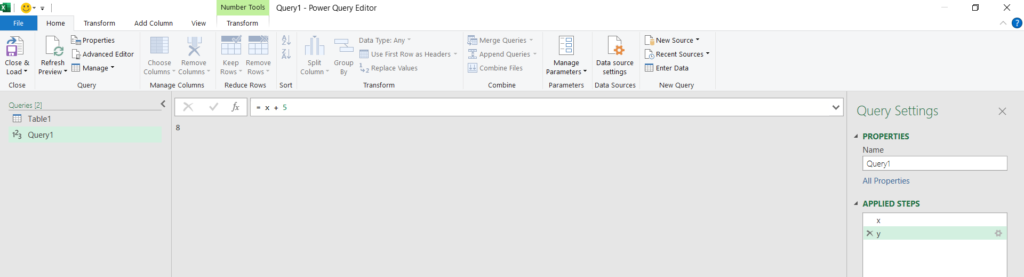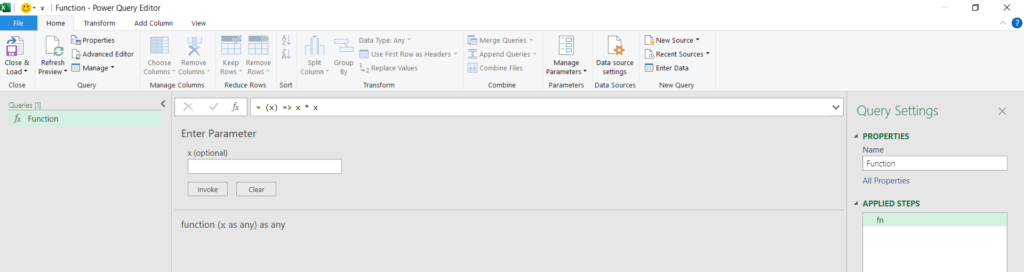Data & Intelligence

# A tour of PowerQuery’s M languageIn a previous post, I introduced PowerQuery and demonstrated how to perform various SQL-like operations. This article gives a tour of PowerQuery’s M language that underlies each query.

## `let` and `in`

If you select a query and click on the “Advanced Editor” button in the Home tab, you’ll see something like this:This is the M language code that constitutes our query. We’ll soon come back to the above code, but for now, let’s gain a basic understanding of how M works.

The first thing to know about M is that most M scripts are of the form `let ... in ...`. In such a script, intermediate computations happen inside the `let` statement, and the content after `in` is the script’s return value.

For example, when the M code

```let
x = 3,
y = x + 5
in
y```

is the script underlying a query, then that query appears as follows in the GUI:Interestingly enough, it is not actually necessary for a script to contain the keywords `let` and `inSo` long as the only content in the script evaluates to a value. For instance,

`x = 5`

is a perfectly valid M script!

So, it is more accurate to say that

• The contents of every M script must evaluate to a value.

• `let ... in ...` evaluates the content after `in`. Therefore, since `let ... in ...` evaluates to a value, any script may be of the form `let ... in ...` .

We should also note that one can place the code of the form `x = let ... in ...` within any existing `let` block, and then make use of `x`!

### `let ... in ...` Vs. `select ... from ...`

In my opinion, the `let ... in ...` syntax doesn’t really make much sense. I think the M language would make much more sense if there were no `let` nor `in`And every script simply returned the value of its last line.

It seems to me that`let ... in ...` is supposed to evoke connotations with SQL’s `select ... from ...`. Comparisons between `let ... in ...` and `select ... from ...` quickly break down, though:

• The data source in a SQL query is specified in the `from` clause, while the data source of a `let ... in ...` statement typically appears in the `let` clause.

• The result set of a SQL query is determined primarily from the `select` clause, while the result of a `let ... in ...` statement is whatever comes after `in`.

##### The Future of Big Data

With some guidance, you can craft a data platform that is right for your organization’s needs and gets the most return from your data capital.

Get the Guide

## Autogenerated M code

Now that we have some knowledge about `let ... in ...`We can look at some sample M code that is autogenerated after using the GUI to create a query:

```let
Source = Excel.CurrentWorkbook(){[Name="Table1"]}[Content],
#"Changed Type" = Table.TransformColumnTypes(Source,{{"col1", Int64.Type}, {"col2", type text}, {"col3", type text}}),
#"Filtered Rows" = Table.SelectRows(#"Changed Type", each [col1] = 1 or [col2] = "b")
in
#"Filtered Rows"```

Looking closely at the above code teaches us two important facts about the M language:

1. Variable identifiers can be of the form `#"{string}"`, where `{string}` is any string of characters.

2. The autogenerated M code corresponding to each “step” in a PowerQuery query references the previous step. (E.g., when computing `#"Changed Type"`, we pass `Source` to `Table.TransformColumnTypes()`).

If we consult the M documentation for any of the functions (`Excel.CurrentWorkbook()`, `Table.TransformColumnTypes()`, `Table.SelectRows()`) in the above, we also see that

1. The objects that represent each “step” in a query are of type `table`.

## M data types

• The Microsoft documentation describes M as having the following primitive types: `binary`, `date`, `datetime`, `datetimezone`, `duration`, `list`, `logical`, `null`, `number`, `record`, `text`, `time`, `type`.

• There are also “abstract types”: `function`, `table`, `any`, and `none`.

• Types in M can be declared as `nullable`.

• Some types represent types ( `type number` and `type text` are such types).

### Lists and records

In M, the basic collection types are lists and records. Lists and records are 0-indexed.

Lists are essentially “arrays”, and records map string-valued “keys” to “values.” (So records are essentially “dictionaries”/”hashmaps”).

To initialize a list, use code such as `lst = {1, "a", 2, false}`. To initialize a record, use code such as `rec = [key = 1, key2 = "blah"]`. To access the ith element of a list, use `lst{i}`. To get the value associated with key `key` (e.g. `key = "key1"`) in a record `rec`, use `rec[key]`.

## M uses functional programming

In M, we use functional programming constructs in the place of looping constructs. The go-to functional programming construct is the function `List.Transform()`. Given a list `lst` and a function `fn`, `List.Transform(lst, fn)` returns the list that is the result of applying `fn` to each element of `lst`.

The function `List.Generate()` can also be handy. Whenever you can’t think of a good way to solve your problem by using `List.Transform()`And whenever it is actually best to essentially implement a `for` loop, use this code to do so:

`List.Generate(() => 0, each _ < n, each _ + 1, {statement})`

It will execute `{statement}` n times.

## User-defined functions

Writing user-defined functions in M can prove very useful. In my work, I found that I needed to repeat a certain sequence of steps many times. If I were to manually rewrite these steps with the PowerQuery GUI repeatedly, I would drive myself insane and have way too many PowerQuery steps. But, since I created a user-defined function to perform the above task, I was able to perform collapse the above four steps into a single step!

The syntax for defining a custom function uses anonymous function syntax.

`fn = (x) => x * x`

(If you were to evaluate `fn(x)` elsewhere in the script, that invocation `fn(x)` would return `x * x`).

The query whose M script is the above looks like this in the GUI:## Global variables and global functions

When a variable or function is used multiple times in multiple scripts, it is best practice to separate the definition of the variable or function from all of the scripts that use the variable or function. To define a global variable with a value of, say, 5, use the Advanced Editor* to make a query’s M code

`5`

Then, change the name of the query to be the desired identifier for the variable.

Since functions are variables of type `function`, the process for defining a global function is the same. For example, to declare a global function named `fn` that sends `x` to `x * x`, create a query whose name is `fn`, and edit the query’s M code with the Advanced Editor* so that it is

`(x) => x * x`

* If you use the smaller editor instead of the Advanced Editor, you will have to prepend an equals `=` to the beginning of your code to avoid errors.

## Accessing the “current” table row

Recall that the function call that implements the equivalent of a general `where` clause looks something like

`Table.SelectRows(#"Changed Type", each [col1] = 1)`

There are a several concepts at play here we glossed over before that deserve explanation.

• Rows of tables are represented as records. If `row` is a record that represents some row of a table, the value in the column `row` of that row is `row[col]`.

• The second argument of `Table.SelectRows()` is a function whose input is a record that represents the “current row” of the table and whose output is a `logical` (i.e. a boolean) that indicates whether or not to include the current row in the result set.

• `_` is a valid variable name in M, and so the function `(_) => fn(_)` is the same as the function `(x) => fn(x)` . For example, the function `(_) => _ * _` is the same as the function `(x) => x * x`.

• The `each` keyword is shorthand for the syntax`(_) =>`.

• Whenever a variable `var` appears in square brackets to the right of an `each`, M interprets `[var]` as meaning `_[var]`. Therefore, an expression such as `each [var]` is the same `(_) => _[var]`.

Knowing all of these things, we see that the above code translates to

`Table.SelectRows(#"Changed Type", (_) => _[col1] = 1)`

Since you might be uncomfortable with using `_` as a variable, let’s consider another equivalent function call:

`Table.SelectRows(#"Changed Type", (row) => row[col1] = 1)`

Here, we understand `(row) => row[col1] = 1` to be the function that takes in a record representing the current row, looks at the value in this record associated with the key `col1`, and returns true whenever that value is equal to `1`. Thus, the above code selects the rows from the table that have a value in column `col1` of `1`.

This site uses Akismet to reduce spam. Learn how your comment data is processed.

##### Ross Grogan-Kaylor

Ross Grogan-Kaylor is a Technical Consultant at Perficient’s Minneapolis office. He enjoys engaging with structural patterns in the syntax and in the high-level ideas of software development.

More from this Author